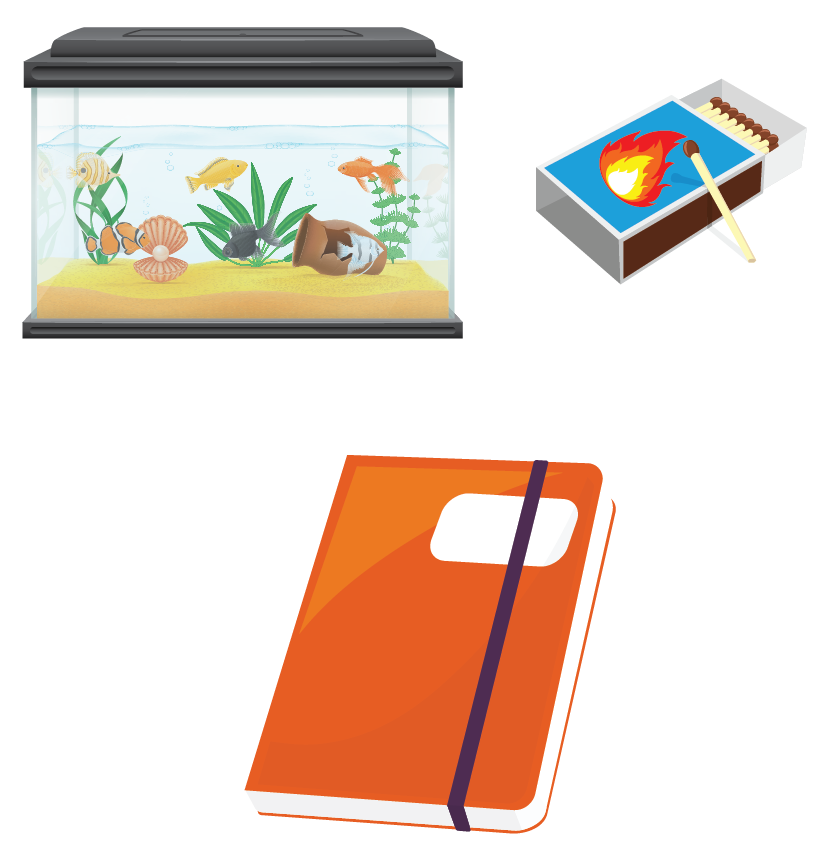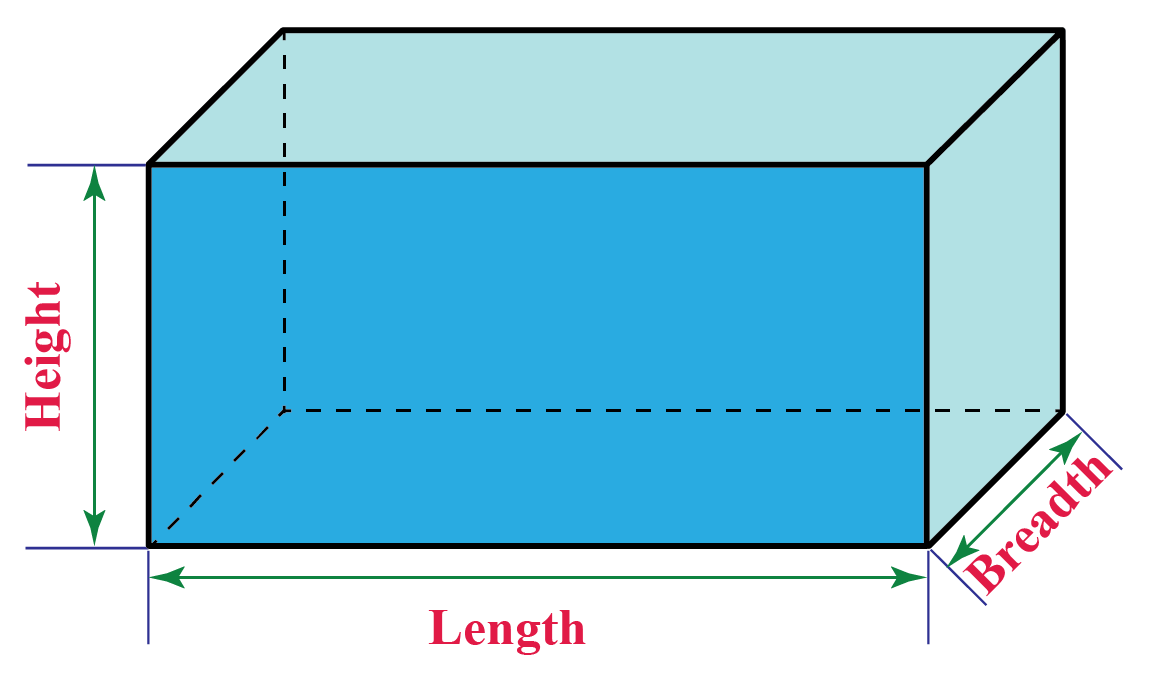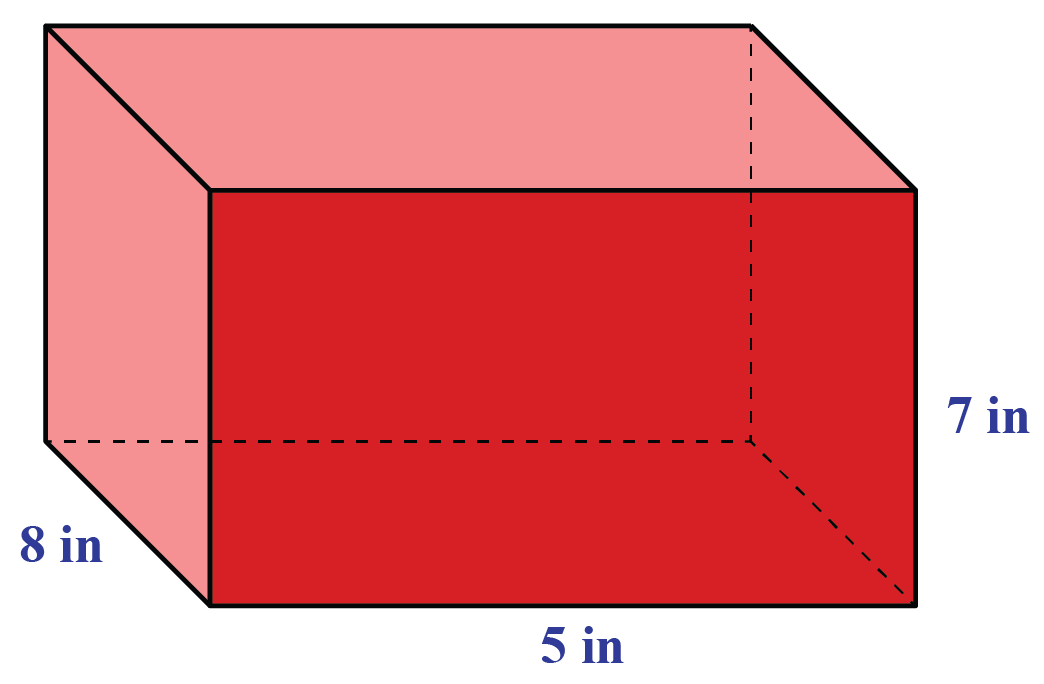# Surface Area of Rectangular Prism

Surface Area of Rectangular Prism
Go back to  'Surface Area'

## Preface

Learn about the surface area of a rectangular prism, the volume of a rectangular prism, right rectangular prism calculation: find a, and right rectangular prism calculation: find v in the concept of Surface Area of Rectangular Prism. Check out the interactive simulations to know more about the lesson and try your hand at solving a few interesting practice questions at the end of the page.

Learn about the surface area of a prism in just 5 minutes!

## Introduction

Let's say there is a box that needs to be gift-wrapped.

You need to calculate the amount of wrapping paper that will be needed to cover it.

First, you will need to know the sum of the areas of the six surfaces of the box (two sides, front, back, top, and bottom). This total area of the six surfaces is called its surface area.Prisms are solids with identical polygon ends and flat parallelogram sides.

The different types of prisms are - triangular prisms, square prisms, rectangular prisms, pentagonal prisms, hexagonal prisms, etc.

Math represents ideas, creative-thinking, and problem-solving. We, at Cuemath, understand this and bridge creative thinking with numbers. At its core, mathematics is simple. And we are good at identifying simplicity.

and experience Cuemath’s LIVE online class with your child.

## What Is the Surface Area of a Rectangular Prism?

Let us first recall a rectangular prism.

A rectangular prism is a three-dimensional shape. It has six faces, and all the faces of the prism are rectangles.

Both the bases of a rectangular prism must be a rectangle. Also, the other lateral faces are rectangles.

For example,

A book, cuboid-shaped aquarium, box, etc.The surface area of a rectangular prism is the total area of all the six faces of the prism.

Add the areas of all the six faces of a rectangular prism to obtain its surface area.

There is a particular type of rectangular prism that is known as a right rectangular prism.

### Right Rectangular Prism

In a right rectangular prism:

• The angles between the base and sides are right angles.
• All 6 faces are rectangles.
• Each corner of the prism is a right angle.
• Each base and top of a rectangular prism is congruent.
• It is also known as a cuboid.

Play with the simulation below to visualize a rectangular prism.

## What Is the General Formula for the Surface Area of a Rectangular Prism?The surface area of a rectangular prism if its length (l), height (h), and breadth (b) are given is:

 Surface Area = $$2(lb+bh+lh)$$

The unit of measurement of the area of the rectangular prism is square units.

### Examples

1. Determine the surface area of a rectangular prism with the following dimensions:

• Length (l) = 8 inches
• Breadth (b) =  5 inches
• Height (h) = 7 inchesThe surface area of the rectangular prism is given by:

\begin{align}2(lb+bh+lh)&=2(8\times 5 + 5\times 7+8\times 7)\\&=2(40+35+56)\\&=2\times 131\\&=262 \text{ inch}^2 \end{align}

Let us also see the formula for the volume of a rectangular prism.

### Volume of Rectangular Prism

The volume of a rectangular prism if its length (l), height (h), and breadth (b) are given is:

 Volume = $$lbh$$Tips and Tricks

• A rectangular prism with all sides equal is called a cube.
• A rectangular prism in which the faces are not perpendicular to each other is called an oblique rectangular prism.
• If all the edges of a rectangular prism are equal, then the surface area of the prism is $$6$$ times the area of each face.
• If all the edges of a rectangular prism are equal to $$l$$, then the volume of the prism is $$l^3$$.

Want to understand the “Why” behind the “What”? Explore the surface area of a rectangular prism with our Math Experts in Cuemath’s LIVE, personalized and interactive online classes.

Make your kid a Math Expert. Book a FREE trial class today!

## How to Calculate the Surface Area of a Rectangular Prism?

Now that we know the formula to calculate the surface area of a rectangular prism, let us now understand how to calculate it with the help of an example.

### Explanation

The dimensions of the box are as follows:

• Length $$l=24 \text{ inches }$$
• Breadth $$b=15 \text{ inches }$$
• Height $$h=20 \text{ inches }$$

Determine the minimum area of the wrapping paper required to wrap the gift.• Step 1: Determine the area of the top and bottom faces of the box.

Since the area of the bottom and the top surfaces are equal, therefore we can twice the area of the top of the box.

\begin{align}2 lb&=2\times (24 \times 15)\\&=2\times 360\\&=720 \text{ inch}^2\end{align}

• Step 2: Next, we determine the area of the side faces of the box.

\begin{align}2 bh&=2\times (15 \times 20)\\&=2\times 300\\&=600 \text{ inch}^2\end{align}

\begin{align}2 lh&=2\times (24 \times 20)\\&=2\times 480\\&=960 \text{ inch}^2\end{align}

• Step 3: Add the areas of all the six faces to determine the total surface area of the box.

\begin{align}2(lb+bh+lh)&=2lb+2bh+2lh\\&=720+600+960\\&=2280 \text{ inch}^2\end{align}Think Tank

Two boxes each of dimension $$4 \text{ inch }\times 5 \text{ inch }\times 6 \text{ inch }$$are joined together face to face to make a rectangular prism box.

Determine the surface area of the newly formed box.

CLUEless in Math? Check out how CUEMATH teachers will explain the surface area of a rectangular prism to your kid using interactive simulations and worksheets so they never have to memorize anything in Math again!

Explore Cuemath's live, interactive, and personalized online classes to make your kid a Math Expert. Book a FREE trial class today!

## Solved Examples

 Example 1

Emily needs to buy some cardboard to build a box without a lid 7 feet long, 5 feet wide, and 9 feet high.

How much cardboard should she buy?

Solution

The dimensions of the box are as follows:

• Length $$l=7 \text{ feet}$$
• Breadth $$b=5 \text{ feet}$$
• Height $$h=9 \text{ feet}$$

We will determine the surface area of the open box.

The surface area of the box is:

\begin{align}lb+2bh+2lh&=7\times 5+2\times 5\times 9+2\times 7\times 9\\&=35+90+126\\&=251 \text{ inch}^2\end{align}

 $$\therefore$$ Emily should buy $$251 \text{inch}^2$$ of cardboard.
 Example 2

Kevin wants to determine the height of a rectangular prism with base area $$10 \text{ feet}^2$$ and volume $$40 \text{ feet}^3$$.

Solution

The volume of a rectangular prism is $$40 \text{ feet}^3$$.

The base area is given by:

$lb=10\text{feet}^2$

The height of the prism is:

\begin{align}\text{Volume}&=40\\lbh&=40\lb)\times h&=40\\10\times h&=40\\h&=4\text{ feet}\end{align}  \(\therefore Height of the prism is $$4 \text{feet}$$
 Example 3

Jacob used $$216\text{ inch}^2$$ of wrapping paper to pack a cubic box.

Determine the length of each edge of the box.

Solution

Since the box is cubic, therefore the length of each edge $$a$$ is equal.

The surface area of a cubic box is given by:

\begin{align}\text{Surface Area}&=216\text{ inch}^2\\6a^2&=216\\a^2&=\dfrac{216}{6}\\a^2&=36\\a&=6\text{ inch}\end{align}

 $$\therefore$$ Length of each edge is $$6\text{ inch}$$

## Practice Questions

Here are a few activities for you to practice.

## Conclusion

We hope you enjoyed learning about the surface area of a rectangular prism with the simulations and practice questions. Now, you will be able to easily solve problems on the surface area of a rectangular prism, the volume of a rectangular prism, right rectangular prism calculation: find a, and right rectangular prism calculation: find v.

You can also simplify this topic with our Math Experts in Cuemath’s LIVE and interactive online classes. Book a FREE trial class today!

## 1. How do you construct a rectangular prism?

A rectangular prism can be constructed by joining six rectangles in a way such that the opposite rectangles are parallel to each other.

## 2. What is the unit for the surface area of a rectangular prism?

The unit of measurement for the surface area of a rectangular prism is square units.

## 3. How do you find the diagonals of a rectangular prism?

The diagonal $$d$$ of a rectangular prism of length $$l$$, breadth $$b$$ and height $$h$$ is given by:

$$d=\sqrt{l^2+b^2+h^2}$$

More Important Topics
Numbers
Algebra
Geometry
Measurement
Money
Data
Trigonometry
Calculus